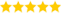Must-Know How to Input a List in Python & How to Master It

# Must-Know How to Input a List in Python & How to Master It

Last updated on 22nd Jun 2020, Blog, General

Dheena (Sr Technical Project Manager - Python )

He is Highly Experienced in Respective Technical Domain with 11+ Years, Also He is a Respective Technical Trainer for Past 5 Years & Share's This Important Articles For us.(5.0) | 19623 Ratings 760
• As you might already know, in order to accept an input from the user in Python, we can make use of the input() function. When used, it enables the programmer to accept either a string, integer or even a character as an input from the user. But when it comes to accepting a list as an input, the approach we follow is slightly different.
• This how to input a List in Python article, will address main areas of concern

Accept a list of number as an input in Python

Take a look at the example program below, which accepts a list of numbers as an input in Python.

• input_string = input(“Enter a list element separated by space “)
• list  = input_string.split()
• print(“Calculating sum of element of input list”)
• sum = 0
• for num in list:
• sum += int (num)
• print(“Sum = “,sum)

Output

Enter a list element separated by space 2 4 6 9

Calculating sum of element of input list

Sum =  20

Analysis

Now let us breakdown the program and see how the workings behind it.

• As you already know, whenever we use the input() function in Python, it converts the user input into a string. Therefore in the above program, we accepted a list element from a user in the form of a string which is separated by spaces.
• One thing to note here, is that you have the ability to accept a string separated by the operator comma (,) as well. But in this situation you need to make use of the split() function in order to pass the argument as well as the separator in the Python program.
• If you look closely, you will find that we have made use of the function input_string.split() to split the input string separated by spaces from the user and converted them into individual elements to be added into a list.
• We have also made use of the For loop and converted every element into an integer to calculate its sum.

Accept a List of Strings from the User

• input_string = input(“Enter family members separated by comma “)
• family_list  = input_string.split(“,”)
• print(“Printing all family member names”)
• for name in family_list:
• print(name)

When the above program is run, the output will look something like this.

Enter family members separated by comma: Julius,Mark,John

Printing all family member names

• Juluis
• Mark
• John
• Analysis
• Similar to the earlier example, we accepted an input list from the user in the form of a string separated by commas.
• We have made use of the input_string.split(“,”) function to split the string separated by commas and convert it into a list of string to be used in the program.
• We used a for loop and printed out all family names in order, as you can see in the output shared above.### Best Hands-on Practical Python Training By Top-Rated Instructors

• Instructor-led Sessions
• Real-life Case Studies
• Assignments

Examples

Let us take a look at a couple of other examples to understand how to input a list in Python.

Example 1

• # creating an empty list
• lst = []
• # number of elemetns as input
• n = int(input(“Enter number of elements : “))
• # iterating till the range
• for i in range(0, n):
• ele = int(input())
• lst.append(ele) # adding the element
• print(lst)

Output

Example 2

• # try block to handle the exception
• try:
• my_list = []
• while True:
• my_list.append(int(input()))
• # if input is not-integer, just print the list
• except:
• print(my_list)

Output